- 如果训练时间过长，一种可能的做法是，增大 minibatch 的大小，以减少训练样本的方差(variance)，有助于算法收敛.

 - 如果得到 NaN 预测结果，可能是算法接收到较大的梯度值，导致内存溢出(memory overflow). 可以将其视为多次迭代后的连续矩阵乘法. 减小学习率，可能会有主于减少这些值的大小. 减少网络层数，可以减少矩阵乘法的数量. 梯度裁剪(clipping gradients) 可以显式的解决该问题.

## 1. 神经网络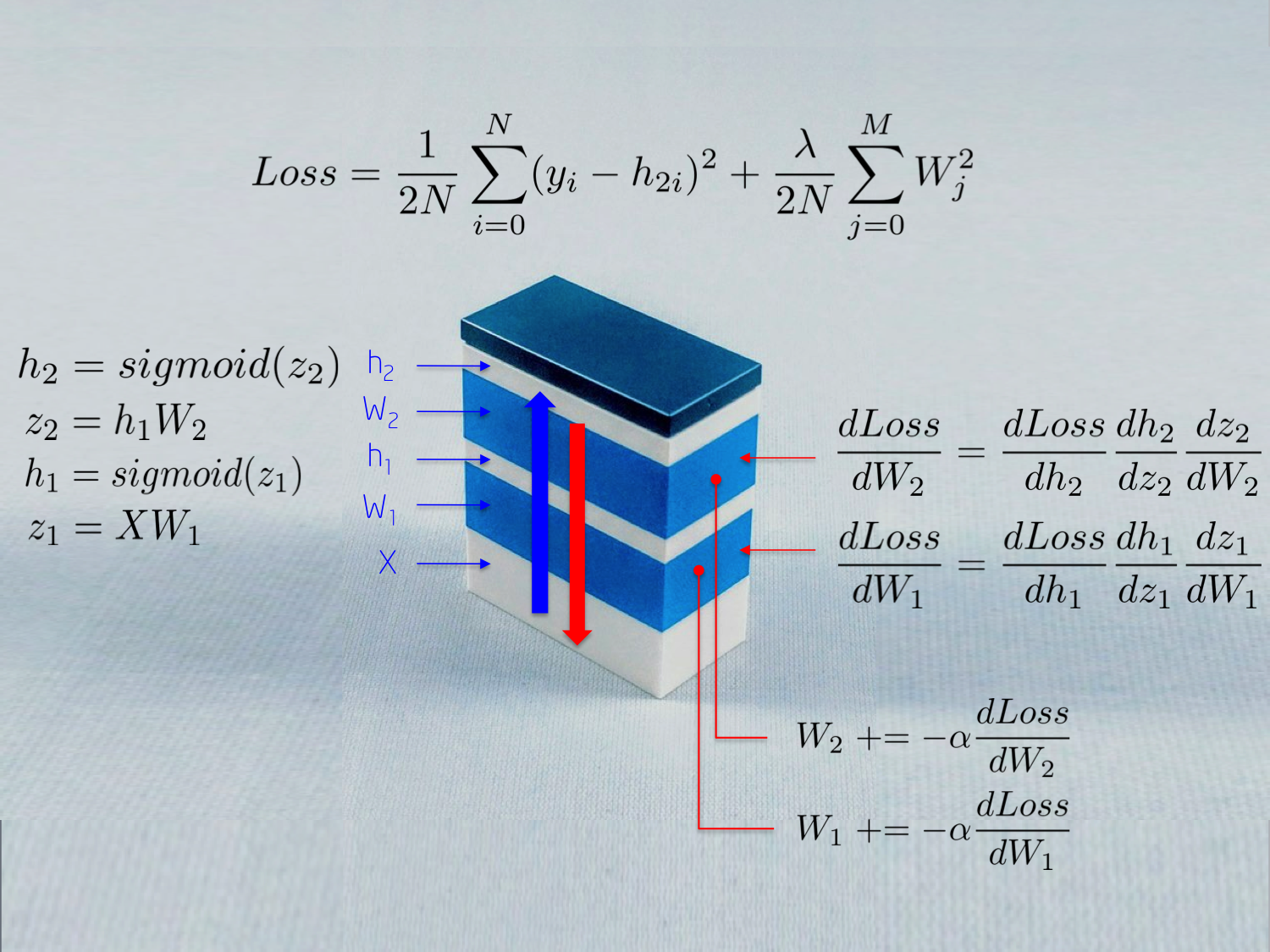- 输入Input X

X 为送入神经网络的原始数据，数据格式为矩阵形式，其中，矩阵的行表示一个数据样本，矩阵的列表示维度.

 - 权重Weights W1

W1 对输入 X 进行映射到第一个隐藏层 h1. 权重 W1 作为线性核.

 - Sigmoid 激活函数

Sigmoid 激活函数用于防止隐藏层 h1 的输出超出范围，并归一化到 [0, 1] 区间. 其输出是一个激活值数组：h1 = Sigmoid(WX).

## 2. XOR 非线性函数实例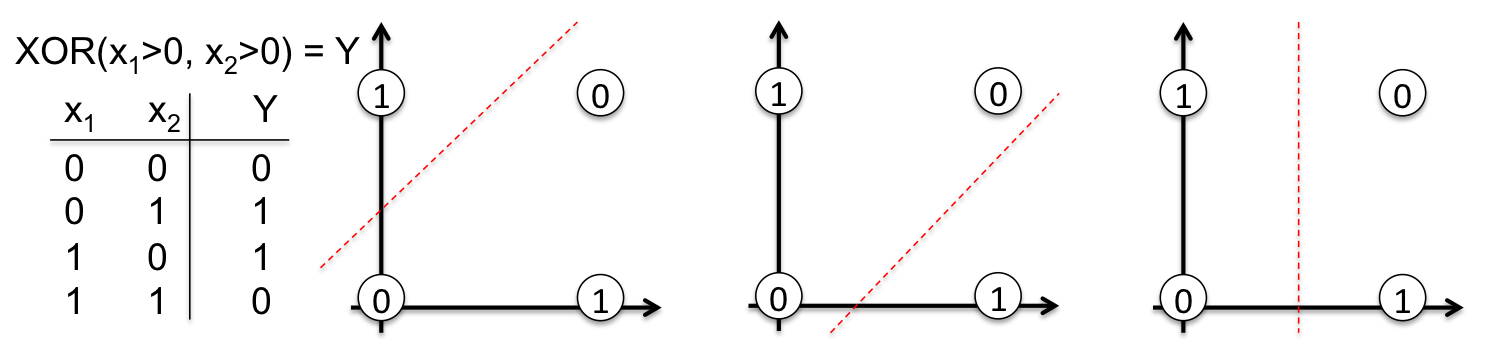- 输入Input X，二维向量；

 - 权重Weights W1，2x3 的矩阵，随机初始化矩阵的元素值.

 - 隐藏层Hidden layer h1，包括三个神经元. 每个神经元的输入是观察值的加权和，如下图的 green 高亮的内积部分：z1=[x1, x2][w1, w2].

 - 权重Weight W2，3x2 的矩阵，随机初始化矩阵的元素值.

 - 输出层Output layer h2，包含两个神经元，因为 XOR 函数返回的值是 0(y1=[0, 1]) 或 1(y2=[1, 0]).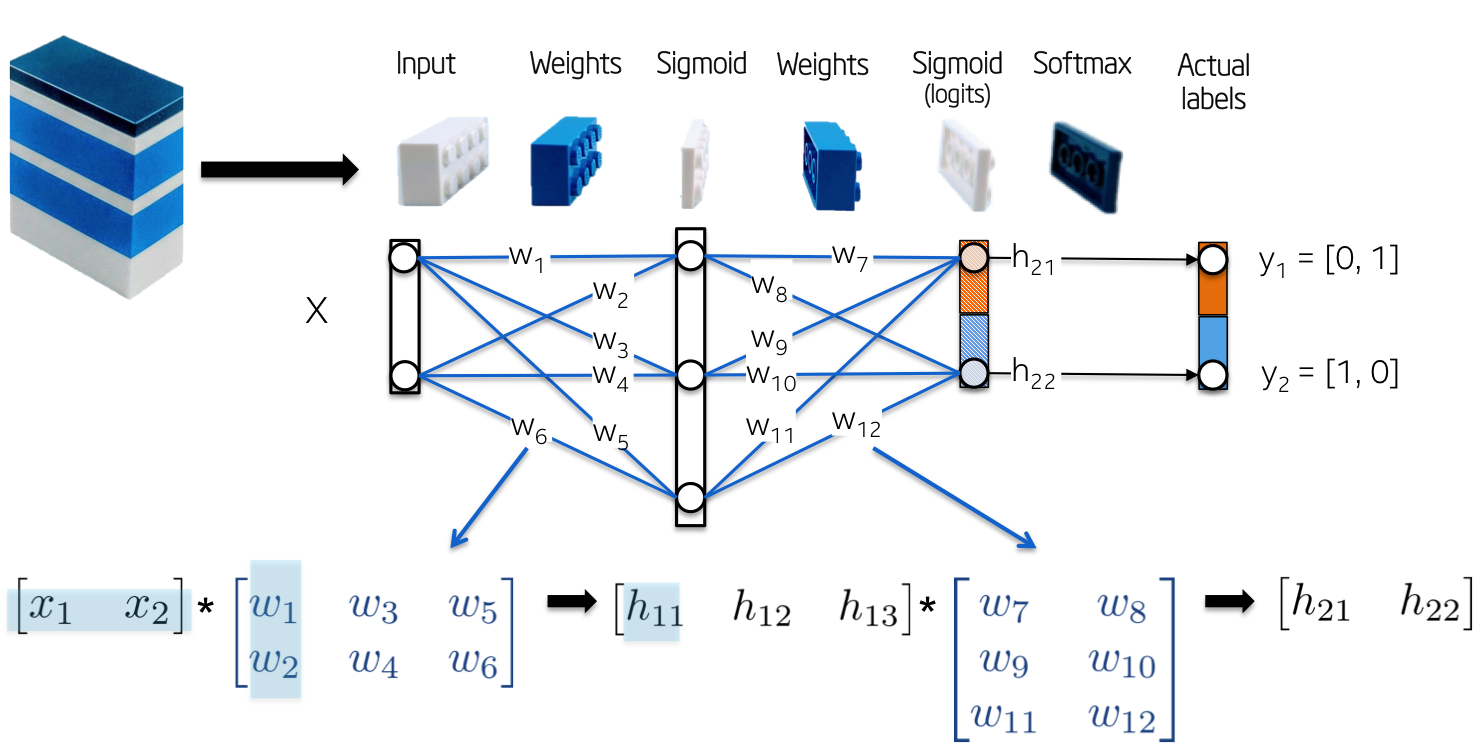BP(Backpropagation) 是用于朝某个方向(gradient)更新权重的方法，其主要通过对批量的标注数据的，计算最小化预定义的误差度量，即损失函数. BP 算法已经被多次重复研究，在反向累计模式中，作为更通用的自动微分(automatic differentiation) 的一种特例.

### 2.1. 网络初始化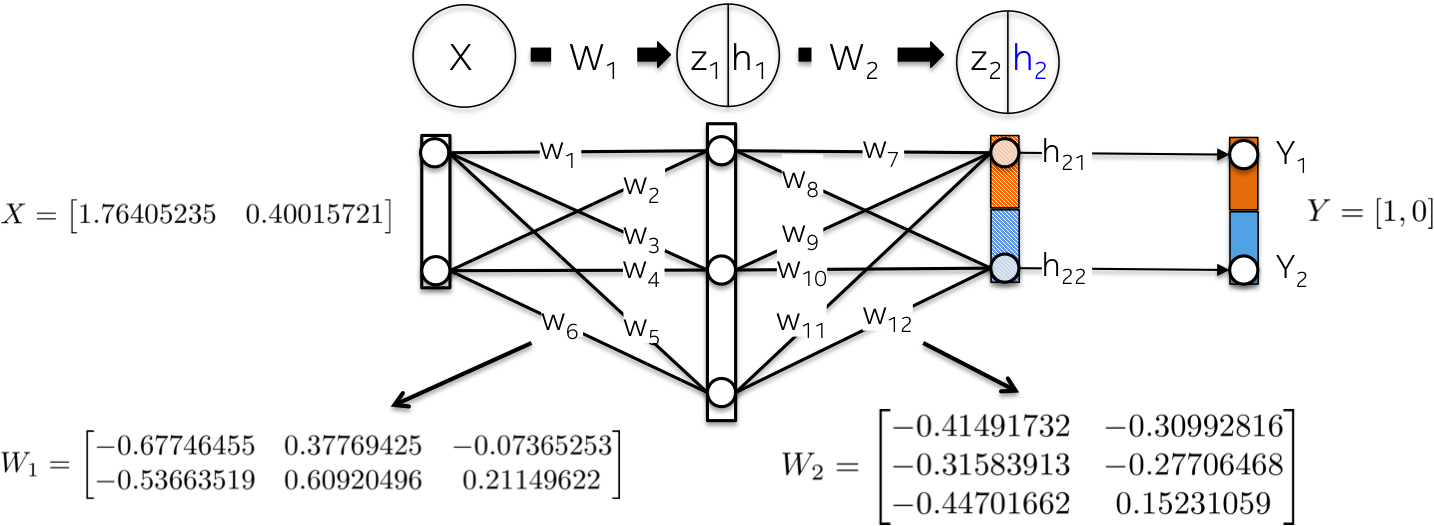### 2.2. 前向计算(Forward)

 - 采用权重 W1 作为 kernel，线性映射输入数据 X：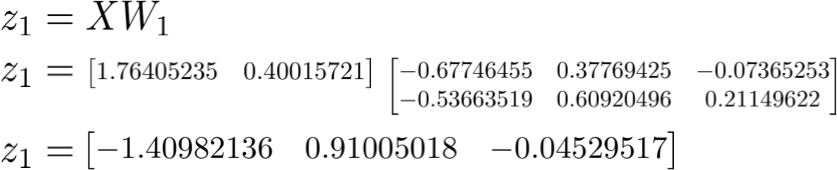- 采用 Sigmoid 函数缩放加权和 z1，以得到第一个隐藏层 h1 的值. 注：原始的 2D 输入向量已经被映射到 3D 空间.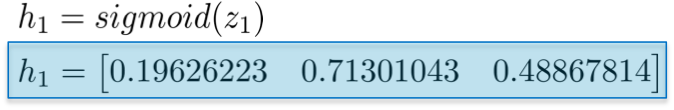- 类似的，对于第二个网络层 h2 进行相同处理. 计算第一个隐藏层的加权和 z2，在这里，第一个隐藏层的输出作为第二个网络层的输入.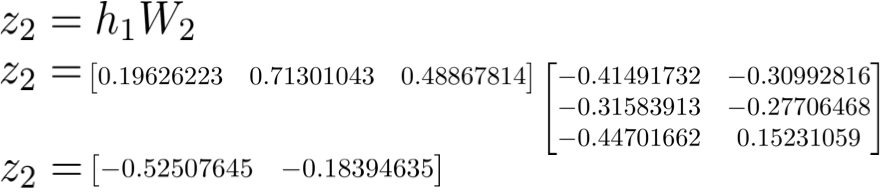- 然后，计算 Sigmoid 激活函数. 向量 [0.37166596 0.45414264] 表示网络关于给定输入 X 所计算得到的 log 概率或者预测向量(log probability or predicted vector).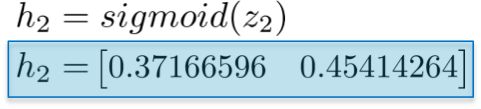- 计算总损失函数(loss)### 2.3. 反向计算(Backward)

 - dLoss/dW2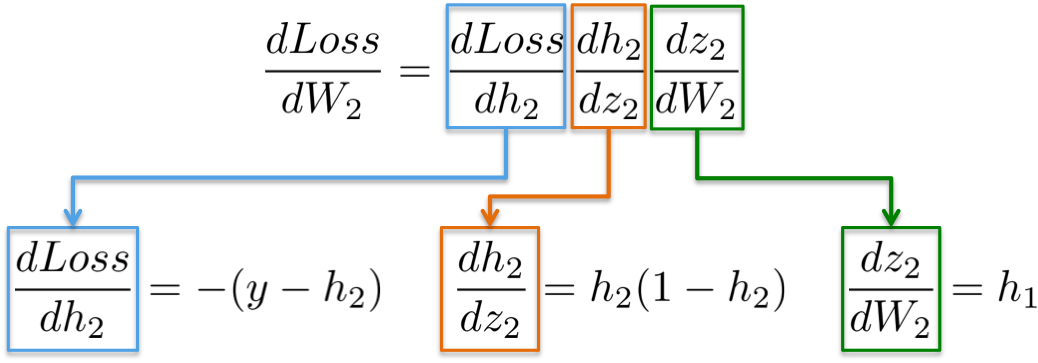Loss = (y-h2)^2dLoss/dW2 = -(y-h2)
h2 = Sigmoid(z2)dh2/dz2 = h2(1-h2)
z2 = h1W2dz2/dW2 = h1
z2 = h1W2dz2/dh1 = W2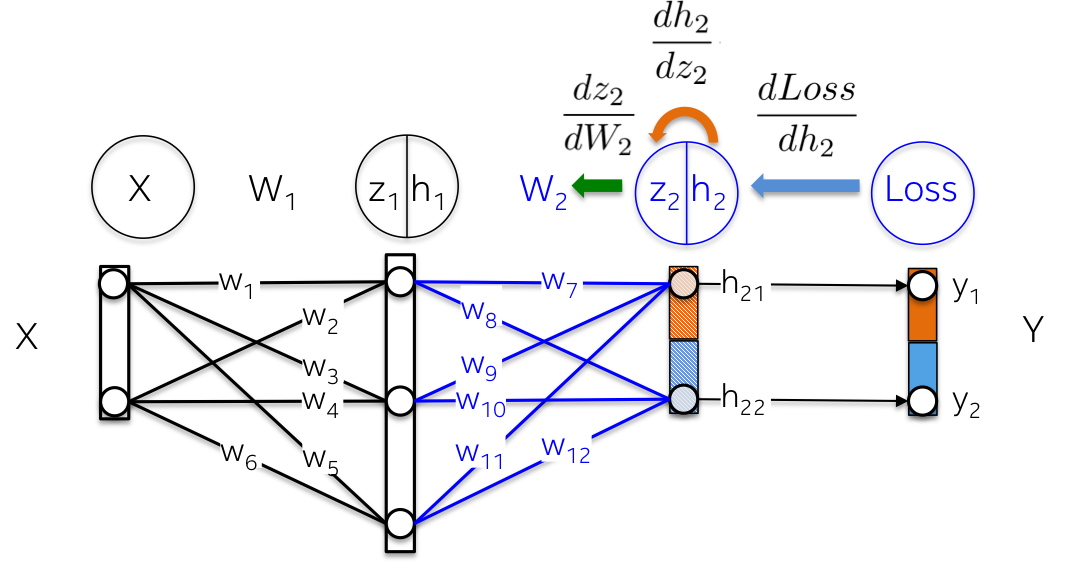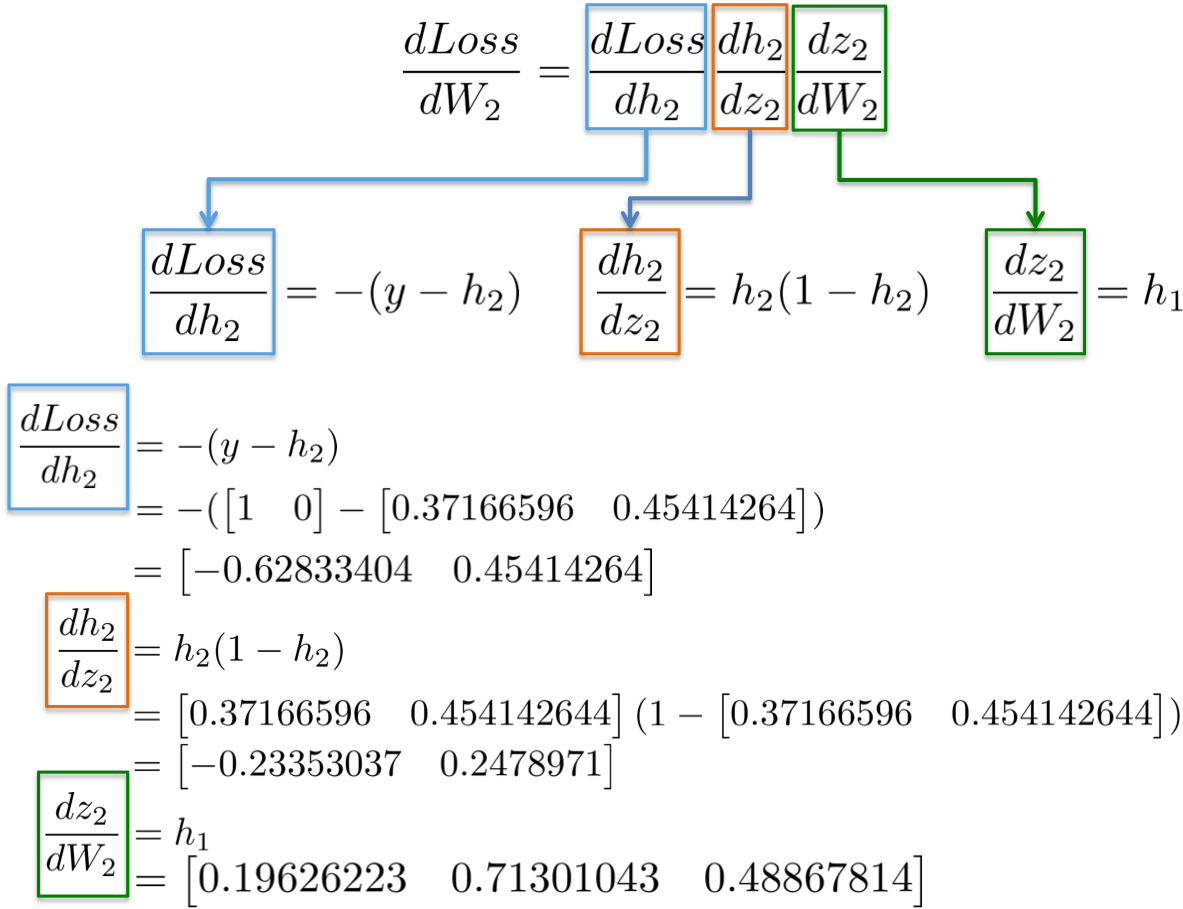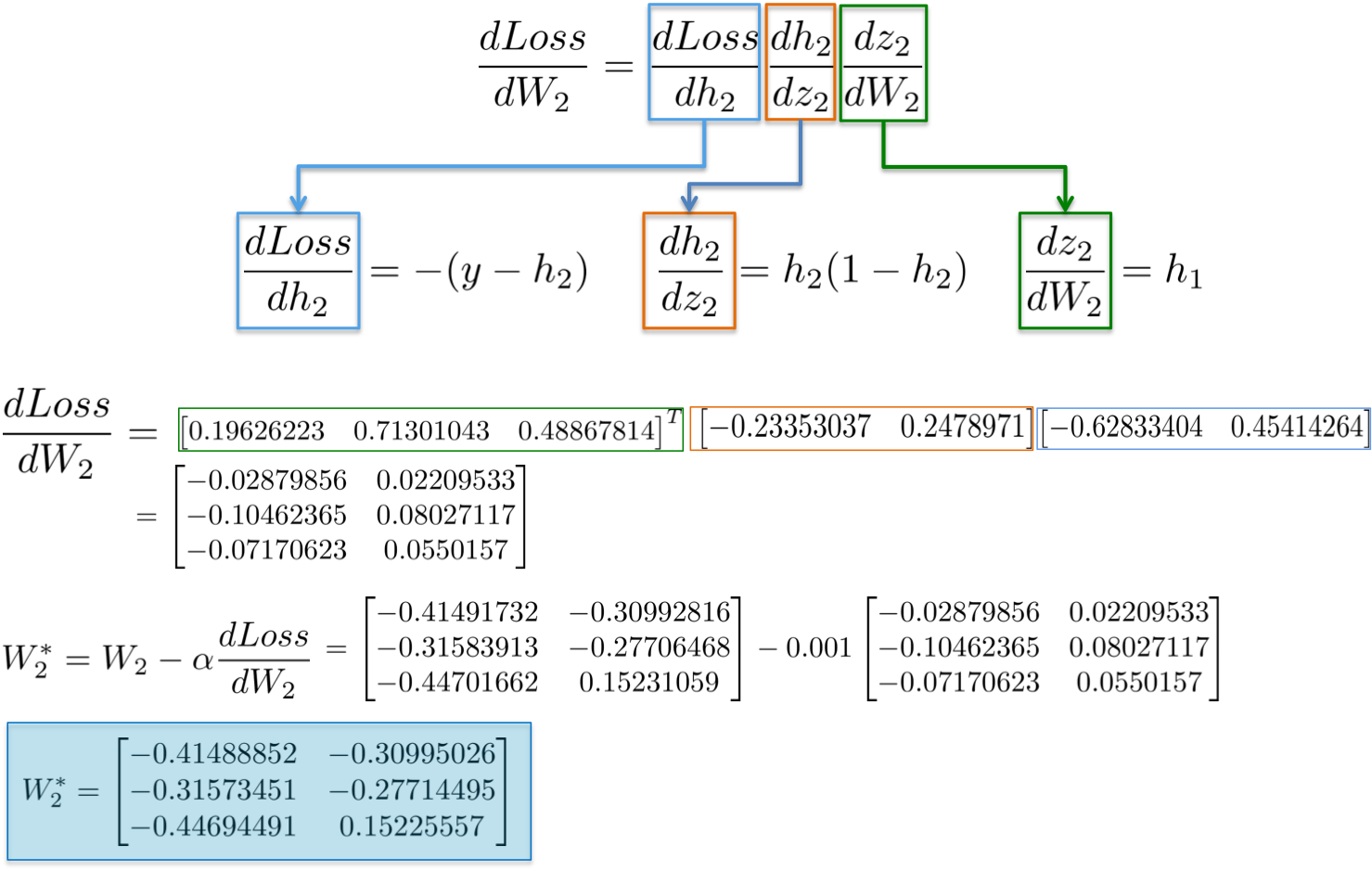- dLoss/dW1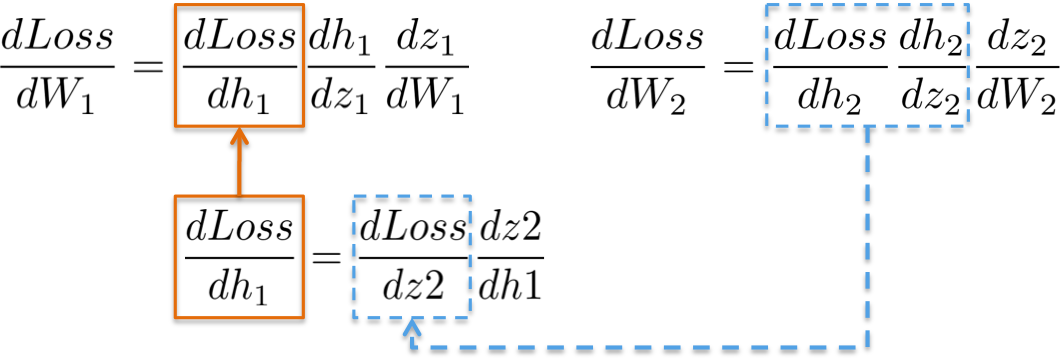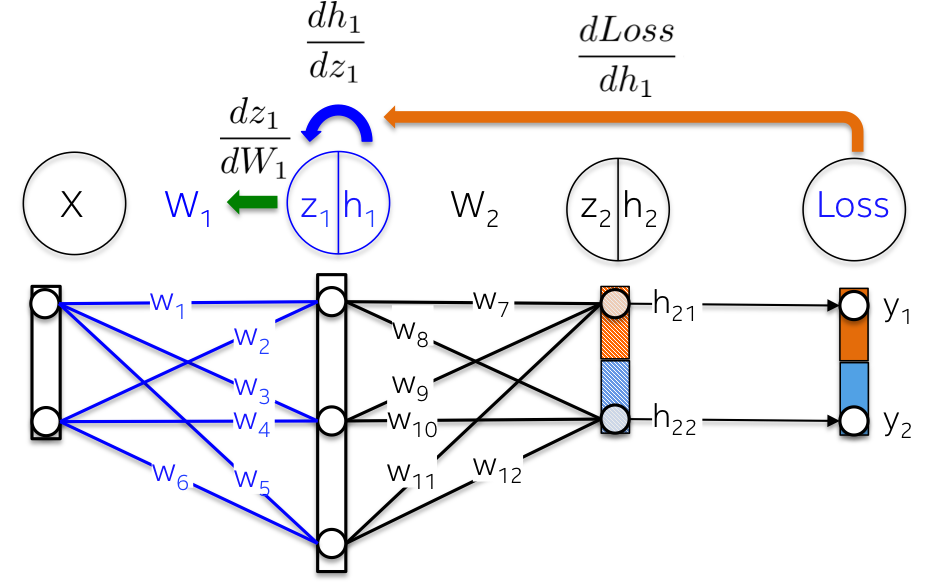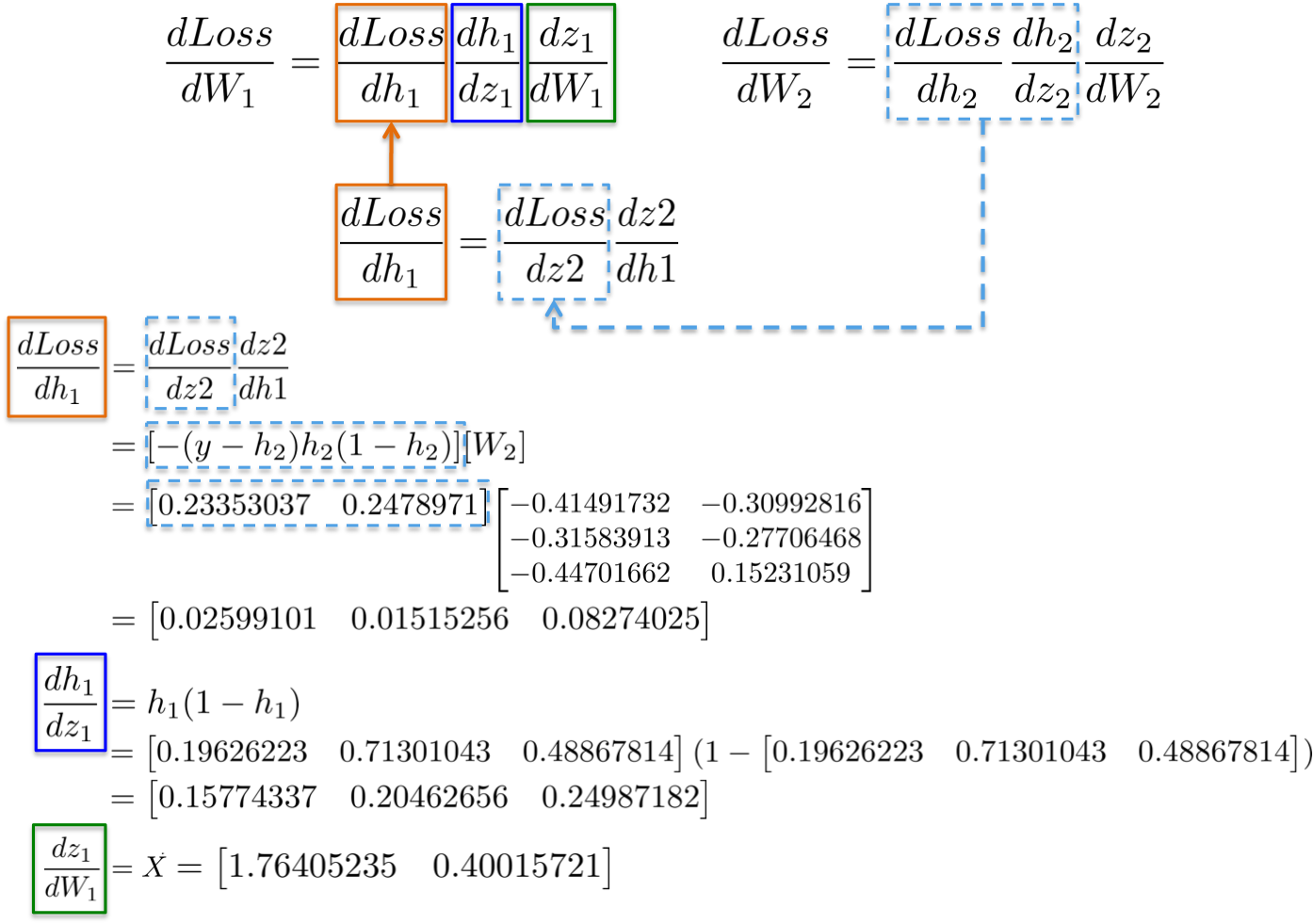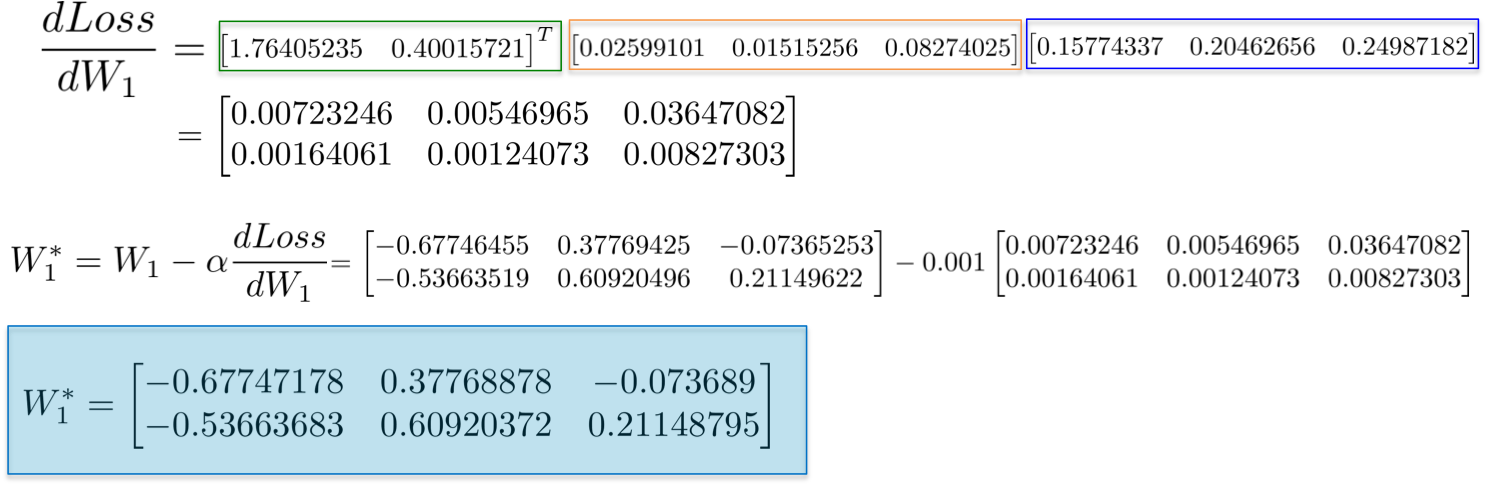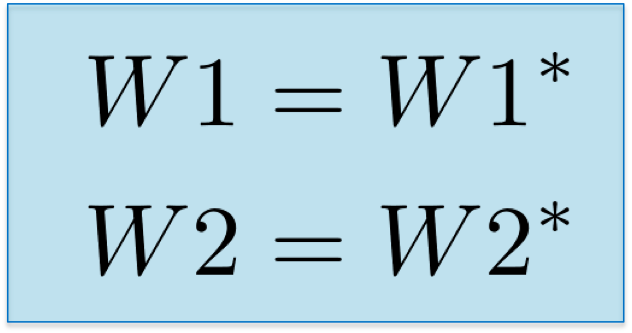## 3. Numpy 实现

#!/usr/bin/env python
import numpy as np
import ipdb
from scratch_mlp import utils
utils.reset_folders()

rng = np.random.RandomState(0)
X = rng.randn(N, 2)
y = np.array(np.logical_xor(X[:, 0] > 0, X[:, 1] > 0), dtype=int)
y = np.expand_dims(y, 1)
y_hot_encoded = []

for x in y:
if x == 0:
y_hot_encoded.append([1,0])
else:
y_hot_encoded.append([0, 1])
return X, np.array(y_hot_encoded)

def sigmoid(z, first_derivative=False):
if first_derivative:
return z*(1.0-z)
return 1.0/(1.0+np.exp(-z))

def tanh(z, first_derivative=True):
if first_derivative:
return (1.0-z*z)
return (1.0-np.exp(-z))/(1.0+np.exp(-z))

def inference(data, weights):
h1 = sigmoid(np.matmul(data, weights))
logits = np.matmul(h1, weights)
probs = np.exp(logits)/np.sum(np.exp(logits), axis=1, keepdims=True)
return np.argmax(probs, axis=1)

def run():
#size of minibatch: int(X.shape)
N = 50
input_dim = int(X.shape)
hidden_dim = 10
output_dim = 2
num_epochs = 1000000
learning_rate= 1e-3
reg_coeff = 1e-6
losses = []
accuracies=[]

#--------------------------------------
# Initialize weights:
np.random.seed(2017)
w1 = 2.0*np.random.random((input_dim, hidden_dim))-1.0      #w0=(2,hidden_dim)
w2 = 2.0*np.random.random((hidden_dim, output_dim))-1.0     #w1=(hidden_dim,2)

#Calibratring variances with 1/sqrt(fan_in)
w1 /= np.sqrt(input_dim)
w2 /= np.sqrt(hidden_dim)
for i in range(num_epochs):
index = np.arange(X.shape)[:N]
#is want to shuffle indices: np.random.shuffle(index)

#----------------------------------
# Forward step:
h1 = sigmoid(np.matmul(X[index], w1))                   #(N, 3)
logits = sigmoid(np.matmul(h1, w2))                     #(N, 2)
probs = np.exp(logits)/np.sum(np.exp(logits), axis=1, keepdims=True)
h2 = logits

#-------------------------------------
# Definition of Loss function: mean squared error plus Ridge regularization
L = np.square(y[index]-h2).sum()/(2*N) + reg_coeff*(np.square(w1).sum()+np.square(w2).sum())/(2*N)

losses.append([i,L])

#---------------------------------------
# Backward step: Error = W_l e_l+1 f'_l
#       dL/dw2 = dL/dh2 * dh2/dz2 * dz2/dw2
dL_dh2 = -(y[index] - h2)                               #(N, 2)
dh2_dz2 = sigmoid(h2, first_derivative=True)            #(N, 2)
dz2_dw2 = h1                                            #(N, hidden_dim)
dL_dw2 = dz2_dw2.T.dot(dL_dh2*dh2_dz2) + reg_coeff*np.square(w2).sum()

#dL/dw1 = dL/dh1 * dh1/dz1 * dz1/dw1
#       dL/dh1 = dL/dz2 * dz2/dh1
#       dL/dz2 = dL/dh2 * dh2/dz2
dL_dz2 = dL_dh2 * dh2_dz2                               #(N, 2)
dz2_dh1 = w2                                            #z2 = h1*w2
dL_dh1 =  dL_dz2.dot(dz2_dh1.T)                         #(N,2)x(2, hidden_dim)=(N, hidden_dim)
dh1_dz1 = sigmoid(h1, first_derivative=True)            #(N,hidden_dim)
dz1_dw1 = X[index]                                      #(N,2)
dL_dw1 = dz1_dw1.T.dot(dL_dh1*dh1_dz1) + reg_coeff*np.square(w1).sum()

w2 += -learning_rate*dL_dw2
w1 += -learning_rate*dL_dw1
if True: #(i+1)%1000==0:
y_pred = inference(X, [w1, w2])
y_actual = np.argmax(y, axis=1)
accuracy = np.sum(np.equal(y_pred,y_actual))/len(y_actual)
accuracies.append([i, accuracy])

if (i+1)% 10000 == 0:
print('Epoch %d\tLoss: %f Average L1 error: %f Accuracy: %f' %(i, L, np.mean(np.abs(dL_dh2)), accuracy))
save_filepath = './scratch_mlp/plots/boundary/image_%d.png'%i
text = 'Batch #: %d    Accuracy: %.2f    Loss value: %.2f'%(i, accuracy, L)
utils.plot_decision_boundary(X, y_actual, lambda x: inference(x, [w1, w2]),
save_filepath=save_filepath, text = text)
save_filepath = './scratch_mlp/plots/loss/image_%d.png' % i
utils.plot_function(losses, save_filepath=save_filepath, ylabel='Loss', title='Loss estimation')
save_filepath = './scratch_mlp/plots/accuracy/image_%d.png' % i
utils.plot_function(accuracies, save_filepath=save_filepath, ylabel='Accuracy', title='Accuracy estimation')

if __name__ == '__main__':
run()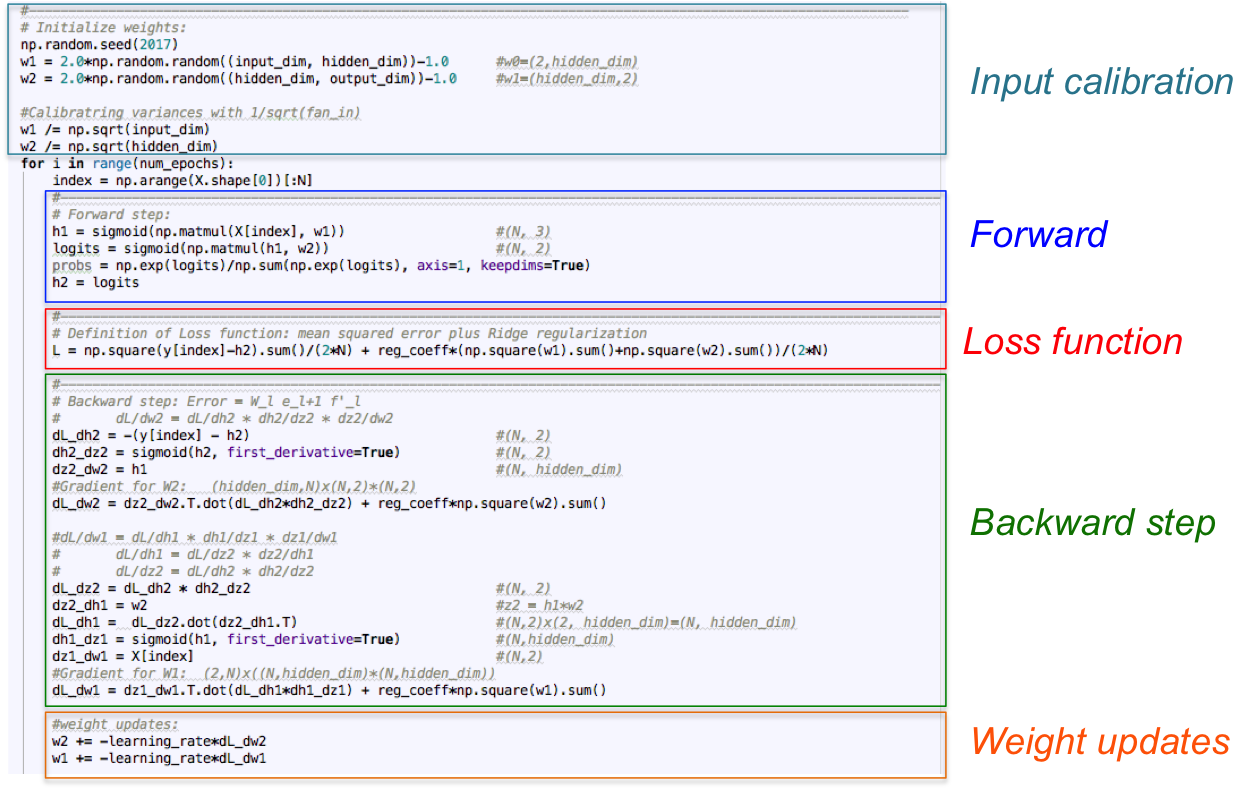### 3.1. 运行

 - 在隐藏层有 3 个神经元的神经网络，具有较弱的表达能力.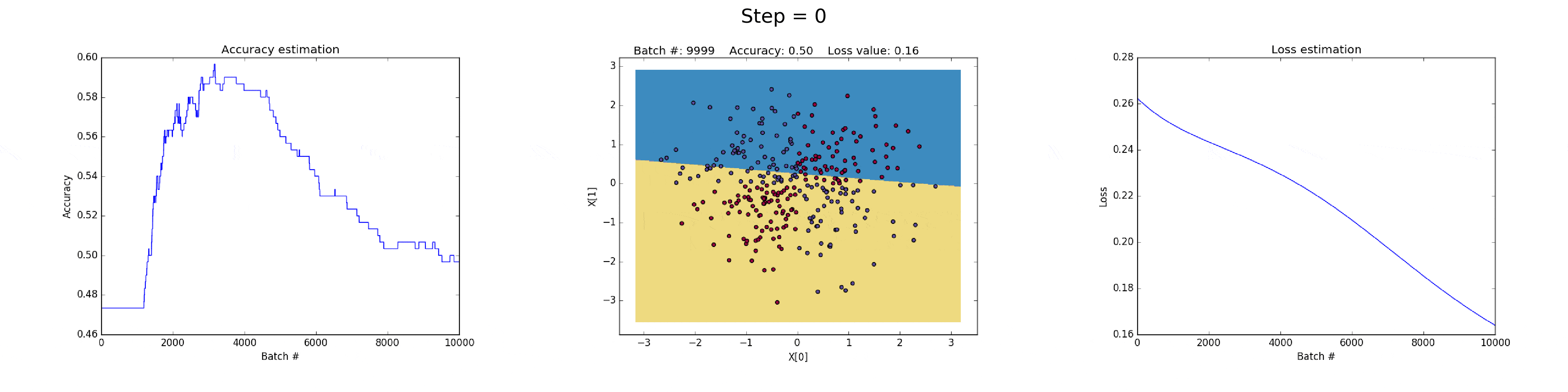- 在隐藏层有 50 个神经元的神经网络，明显增强了模型的特征表达能力.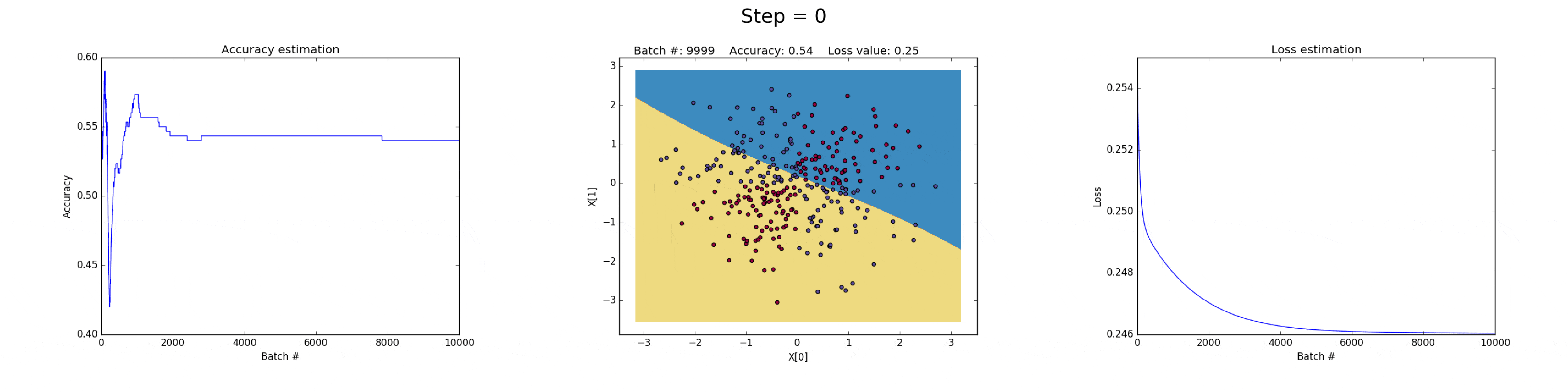Last modification：July 18th, 2019 at 07:22 am